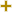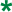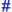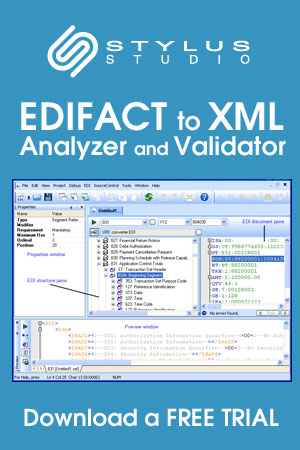# Date or time or period format codeUN/CEFACT Revision 2001A Code ListRepr:an..3
Desc:Code specifying the representation of a date, time or period.
This table also occurs in the following versions of this standard:
D00A, D00B, D01A, D01B, D01C, D02A, D02B, D03A, D03B, D04A, D04B, D93A, D94A, D94B, D95A, D95B, D96A, D96B, D97A, D97B, D98A, D98B, D99A, D99B

2DDMMYY
Calendar date: D = Day; M = Month; Y = Year.
3MMDDYY
Calendar date: M = Month; D = Day; Y = Year.
4DDMMCCYY
Calendar date C=Century; Y=Year; M=Month; D=Day.
5DDMMCCYYHHMM
Calendar date and time: C=Century; Y=Year; M=Month; D=Day; H=Hour; M=Minute.
101YYMMDD
Calendar date: Y = Year; M = Month; D = Day.
102CCYYMMDD
Calendar date: C = Century ; Y = Year ; M = Month ; D = Day.
103YYWWD
Calendar week day: Y = Year ; W = Week ; D = Day Week number 01 is always first week of January Day number 1 is always Monday.
105YYDDD
Calendar day: Y = Year ; D = Day January the first = Day 001 Always start numbering the days of the year from January 1st through December 31st.
106MMDD
Day of a month: M = Month; D = Day.
107DDD
Day's number within a specific year: D = Day.
108WW
Week's number within a specific year: W = Week.
109MM
Month's number within a specific year: M = Month.
110DD
Day's number within is a specific month: D = Day.
201YYMMDDHHMM
Calendar date including time without seconds: Y = Year; M = Month; D = Day; H = Hour; M = Minute.
202YYMMDDHHMMSS
Calendar date including time with seconds: Y = Year; M = Month; D = Day; H = Hour; m = Minutes = Seconds.
203CCYYMMDDHHMM
Calendar date including time with minutes: C=Century; Y=Year; M=Month; D=Day; H=Hour; M=Minutes.
204CCYYMMDDHHMMSS
Calendar date including time with seconds: C=Century;Y=Year; M=Month;D=Day;H=Hour;M=Minute;S=Second.
205CCYYMMDDHHMMZHHMM
Calendar date including time and time zone expressed in hours and minutes. ZHHMM = time zone given as offset from Coordinated Universal Time (UTC).
301YYMMDDHHMMZZZ
See 201 + Z = Time zone.
302YYMMDDHHMMSSZZZ
See 202 + Z = Time zone.
303CCYYMMDDHHMMZZZ
See 203 plus Z=Time zone.
304CCYYMMDDHHMMSSZZZ
See 204 plus Z=Time zone.
305MMDDHHMM
Month, day, hours, minutes; M = Month; D = Day; H = Hour; M = Minute.
306DDHHMM
Day, hours, minutes; D = Day; H = Hour; M = Minute.
401HHMM
Time without seconds: H = Hour; m = Minute.
402HHMMSS
Time with seconds: H = Hour; m = Minute; s = Seconds.
404HHMMSSZZZ
See 402 plus Z=Time zone.
405MMMMSS
Time without hours: m=minutes, s=seconds.
406ZHHMM
Offset from Coordinated Universal Time (UTC) where Z is plus (+) or minus (-).
501HHMMHHMM
Time span without seconds: H = Hour; m = Minute;.
502HHMMSS-HHMMSS
Format of period to be given without hyphen.
503HHMMSSZZZ-HHMMSSZZZ
Format of period to be given without hyphen.
600CC
Century.
601YY
Calendar year: Y = Year.
602CCYY
Calendar year including century: C = Century; Y = Year.
603YYS
Semester in a calendar year: Y = Year; S = Semester.
604CCYYS
Semester in a calendar year: C = Century; Y = Year; S = Semester.
608CCYYQ
Quarter in a calendar year: C = Century; Y = Year; Q = Quarter.
609YYMM
Month within a calendar year: Y = Year; M = Month.
610CCYYMM
Month within a calendar year: CC = Century; Y = Year; M = Month.
613YYMMA
Format of period to be given without hyphen (A = ten days period).
614CCYYMMA
Format of period to be given without hyphen (A = ten days period).
615YYWW
Week within a calendar year: Y = Year; W = Week 1st week of January = week 01.
616CCYYWW
Week within a calendar year: CC = Century; Y = Year; W = Week (1st week of January = week 01).
701YY-YY
Format of period to be given in actual message without hyphen.
702CCYY-CCYY
Format of period to be given in actual message without hyphen.
703YYS-YYS
Format of period to be given without hyphen.
704CCYYS-CCYYS
Format of period to be given in actual message without hyphen.
705YYPYYP
Format of period to be given without hyphen (P = period of 4 months).
706CCYYP-CCYYP
Format of period to be given without hyphen (P = period of 4 months).
707YYQ-YYQ
Format of period to be given without hyphen.
708CCYYQ-CCYYQ
Format of period to be given in actual message without hyphen.
709YYMM-YYMM
Format of period to be given in actual message without hyphen.
710CCYYMM-CCYYMM
Format of period to be given in actual message without hyphen.711
CCYYMMDD-CCYYMMDD
Format of period to be given in actual message without hyphen. Note: 1. This code value will be removed effective with directory D.03B.
713YYMMDDHHMM-YYMMDDHHMM
Format of period to be given in actual message without hyphen.
715YYWW-YYWW
Format of period to be given in actual message without hyphen.
716CCYYWW-CCYYWW
Format of period to be given without hyphen.
717YYMMDD-YYMMDD
Format of period to be given in actual message without hyphen.
718CCYYMMDD-CCYYMMDD
Format of period to be given without hyphen.
719CCYYMMDDHHMM-CCYYMMDDHHMM
A period of time which includes the century, year, month, day, hour and minute. Format of period to be given in actual message without hyphen.
720DHHMM-DHHMM
Format of period to be given without hyphen (D=day of the week, 1=Monday; 2=Tuesday; ... 7=Sunday).
801Year
To indicate a quantity of years.
802Month
To indicate a quantity of months.
803Week
To indicate a quantity of weeks.
804Day
To indicate a quantity of days.
805Hour
To indicate a quantity of hours.
806Minute
To indicate a quantity of minutes.
807Second
To indicate a quantity of seconds.
808Semester
To indicate a quantity of semesters (six months).
809Four months period
To indicate a quantity of four months periods.
810Trimester
To indicate a quantity of trimesters (three months).
811Half month
To indicate a quantity of half months.
812Ten days
To indicate a quantity of ten days periods.
813Day of the week
Numeric representation of the day (Monday = 1).
814Working days
Number of working days.

Change indicators
plus signAn addition.
asteriskAddition/substraction/change to a code entry for a particular data element.
hash or pound signChanges to names.
vertical barChanges to text for descriptions, notes and functions.
minus signA deletion.
letter XMarked for deletion.

Usage indicatorsUsed in batch messages only.Common usage in both batch and interactive messages.Used in interactive messages only.